Question

2. Water flows at a rate of 60 L/s from a main reservoir to a subsidiary...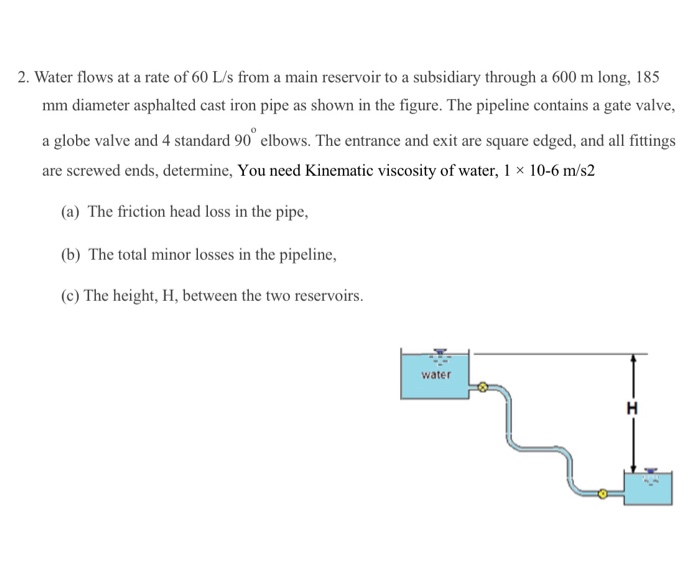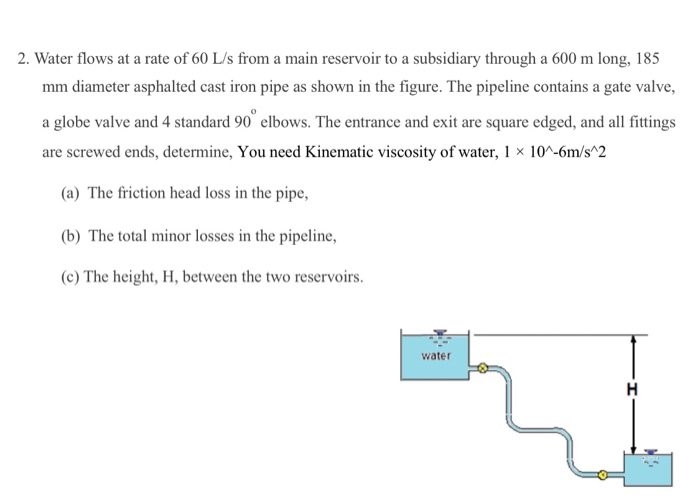2. Water flows at a rate of 60 L/s from a main reservoir to a subsidiary through a 600 m long, 185 mm diameter asphalted cast iron pipe as shown in the figure. The pipeline contains a gate valve, a globe valve and 4 standard 90° elbows. The entrance and exit are square edged, and all fittings are screwed ends, determine, You need Kinematic viscosity of water, 1 x 10-6 m/s2 (a) The friction head loss in the pipe, (b) The total minor losses in the pipeline, (c) The height, H, between the two reservoirs. water H
2. Water flows at a rate of 60 L/s from a main reservoir to a subsidiary through a 600 m long, 185 mm diameter asphalted cast iron pipe as shown in the figure. The pipeline contains a gate valve, a globe valve and 4 standard 90° elbows. The entrance and exit are square edged, and all fittings are screwed ends, determine, You need Kinematic viscosity of water, 1 x 10^-6m/s^2 (a) The friction head loss in the pipe, (b) The total minor losses in the pipeline, (c) The height, H, between the two reservoirs. water H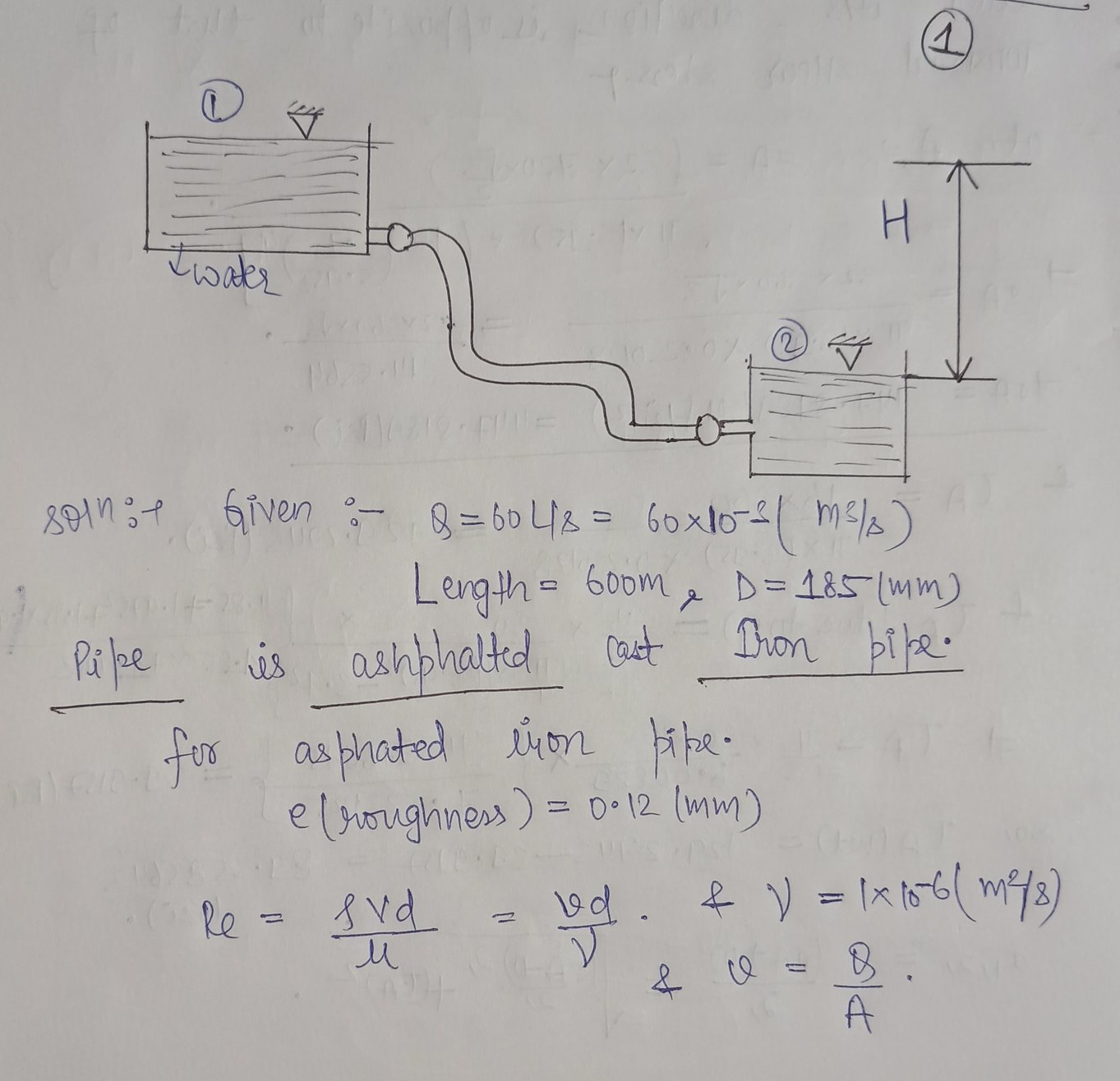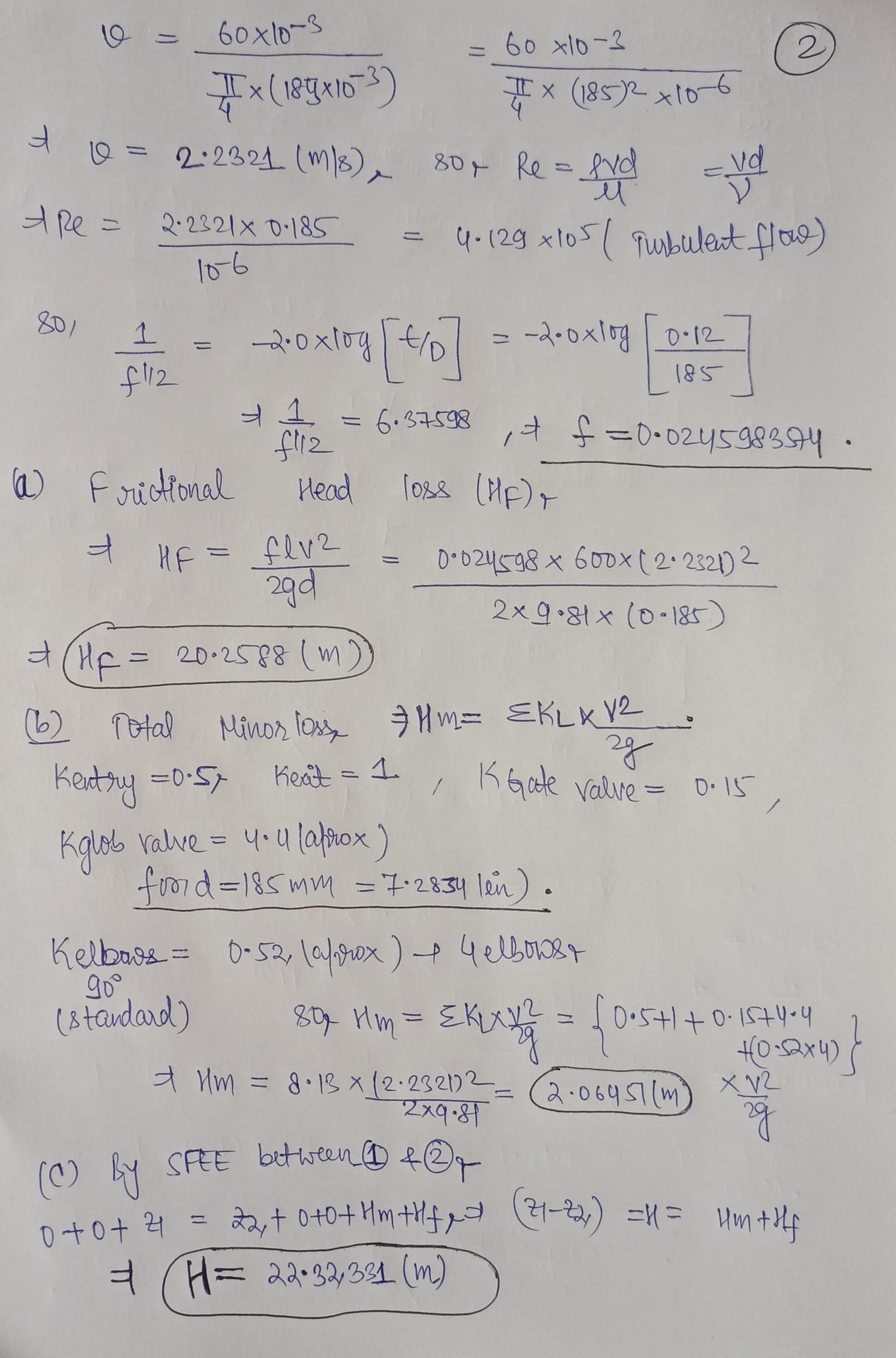Earn Coins

Coins can be redeemed for fabulous gifts.

Similar Homework Help Questions
• Water (density 998 kg/mº, dynamic viscosity 0.001 Pa s) is pumped between two reservoirs at a...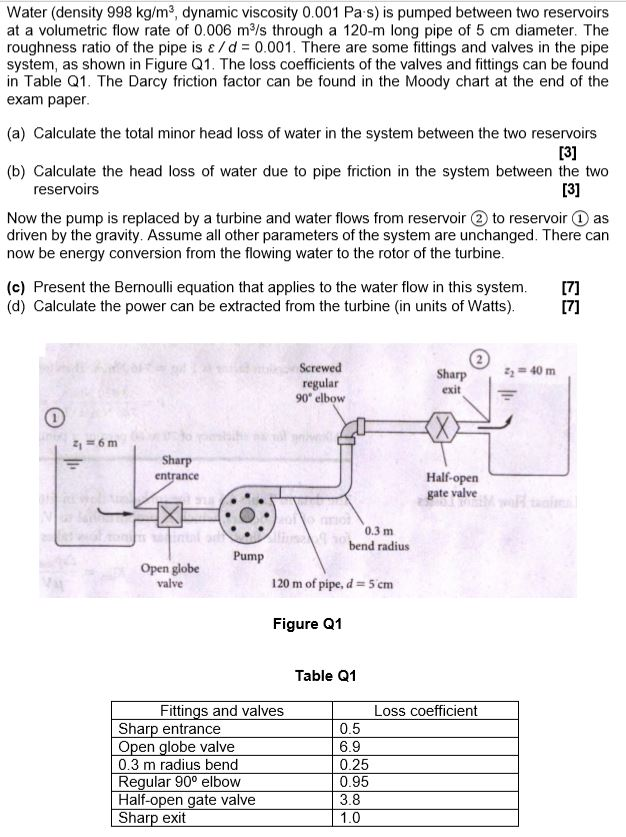Water (density 998 kg/mº, dynamic viscosity 0.001 Pa s) is pumped between two reservoirs at a volumetric flow rate of 0.006 m/s through a 120-m long pipe of 5 cm diameter. The roughness ratio of the pipe is a/d = 0.001. There are some fittings and valves in the pipe system, as shown in Figure Q1. The loss coefficients of the valves and fittings can be found in Table Q1. The Darcy friction factor can be found in the Moody...

• Two water reservoirs A and B are connected to each other through a 35m-long, 2-cm-diameter cast...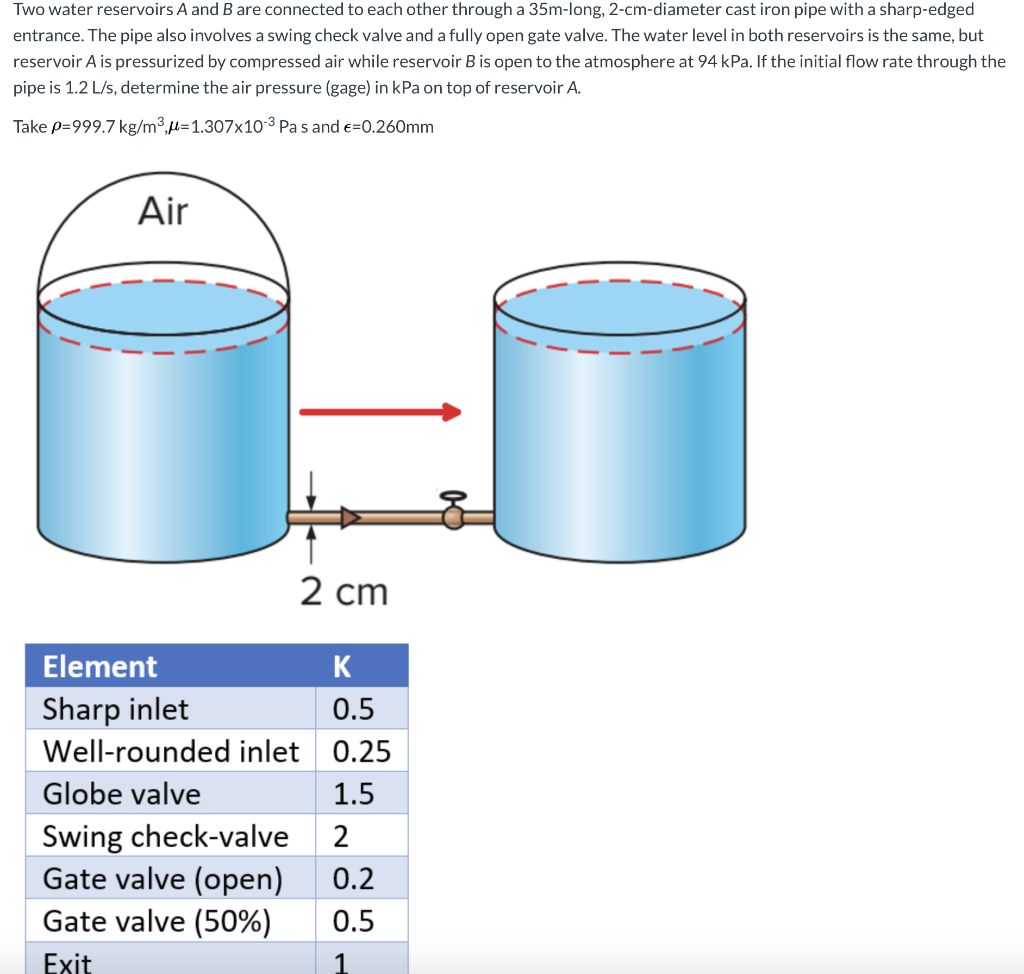Two water reservoirs A and B are connected to each other through a 35m-long, 2-cm-diameter cast iron pipe with a sharp-edged entrance. The pipe also involves a swing check valve and a fully open gate valve. The water level in both reservoirs is the same, but reservoir A is pressurized by compressed air while reservoir B is open to the atmosphere at 94 kPa. If the initial flow rate through the pipe is 1.2 L/s, determine the air pressure (gage)...

• Problem 3 A pipeline delivers water from Reservoir 1 to Reservoir 2 as shown in the following figure. The water levels at Reservoirs 1 and 2 are 50 ft and 20 ft, respectively. A globe valve is in...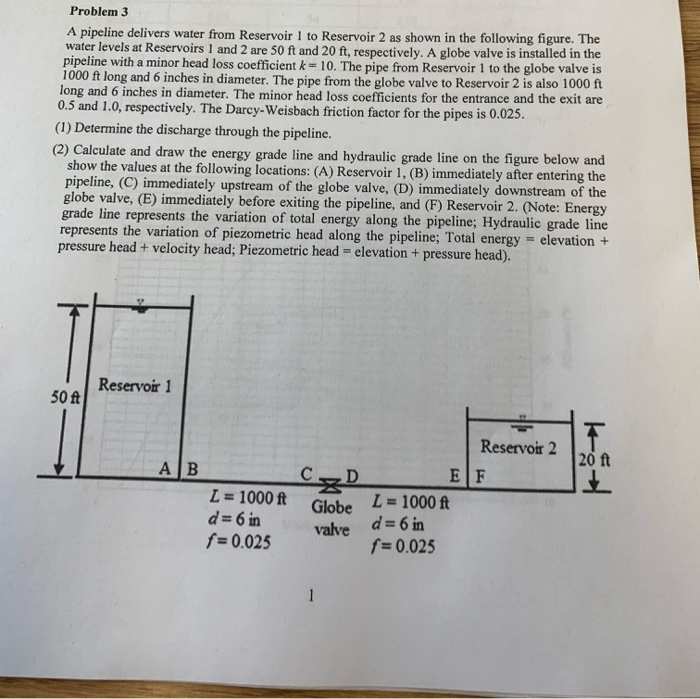Problem 3 A pipeline delivers water from Reservoir 1 to Reservoir 2 as shown in the following figure. The water levels at Reservoirs 1 and 2 are 50 ft and 20 ft, respectively. A globe valve is installed in the pipeline with a minor head loss coefficient k 10. The pipe from Reservoir 1 to the globe valve is 1000 ft long and 6 inches in diameter. The pipe from the globe valve to Reservoir 2 is also 1000 ft...

• can you explain how can I solve this problem? Water at 60°F (15.6°C) (density 62.37 lb/ft3...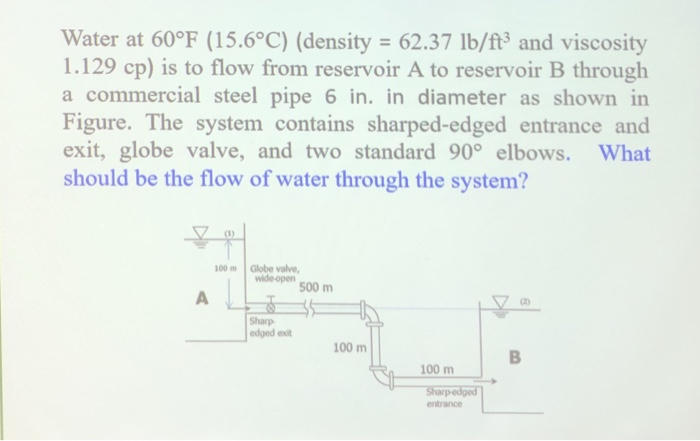can you explain how can I solve this problem? Water at 60°F (15.6°C) (density 62.37 lb/ft3 and viscosity 1.129 cp) is to flow from reservoir A to reservoir B through a commercial steel pipe 6 in. in diameter as shown in Figure. The system contains sharped-edged entrance and exit, globe valve, and two standard 90° elbows. What should be the flow of water through the system? 00m Globe valve wide-open 500 m Sharp edged exit 100 m 100m Sharp-edge entrance

• Water, with density of 1000 kg/m3 and kinematic viscosity of 1.0 x 10- m2/s, is pumped through a ...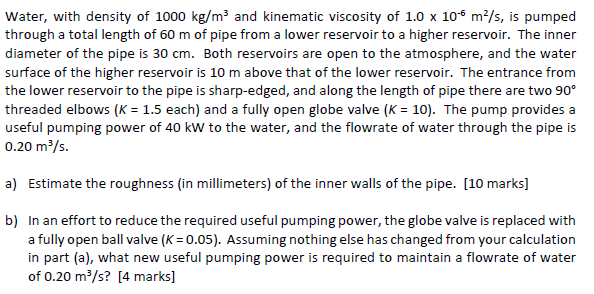Water, with density of 1000 kg/m3 and kinematic viscosity of 1.0 x 10- m2/s, is pumped through a total length of 60 m of pipe from a lower reservoir to a higher reservoir. The inner diameter of the pipe is 30 cm. Both reservoirs are open to the atmosphere, and the water surface of the higher reservoir is 10 m above that of the lower reservoir. The entrance from the lower reservoir to the pipe is sharp-edged, and along the...

• You need to pump water at 20 C from an open reservoir at flow rate of...

You need to pump water at 20 C from an open reservoir at flow rate of 1.4 x 10^-3 m3/s through a pipe with an inside diameter of 0.03m, using a centrifugal pump having an (NPSH)aq =2.5 m and the equivalent length on the suction side is 3m. There are several minor losses: four standar 90 flanged elbows, and fully open flanged globe valve, fully open flanged gate valve, and the Entrance. What is the the maximum distance above the...

• 30 points You need to pump water at 20 °c from an open reservoir at flow...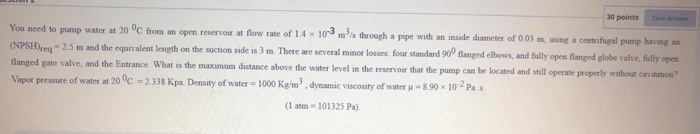30 points You need to pump water at 20 °c from an open reservoir at flow rate of 14 x 10-3 m?s through a pipe with an inside diameter of 0.03 m, using a centrifugal pump having an (NPSH)req = 2.5 m and the equivalent length on the suction side is 3 m. There are several minor losses: four standard 900 flanged elbows, and fully open flanged globe valve, fully open flanged gate valve, and the entrance. What is the...

• Two water reservoirs A and B are connected to each other through a 35m-long, 2-cm-diameter cast...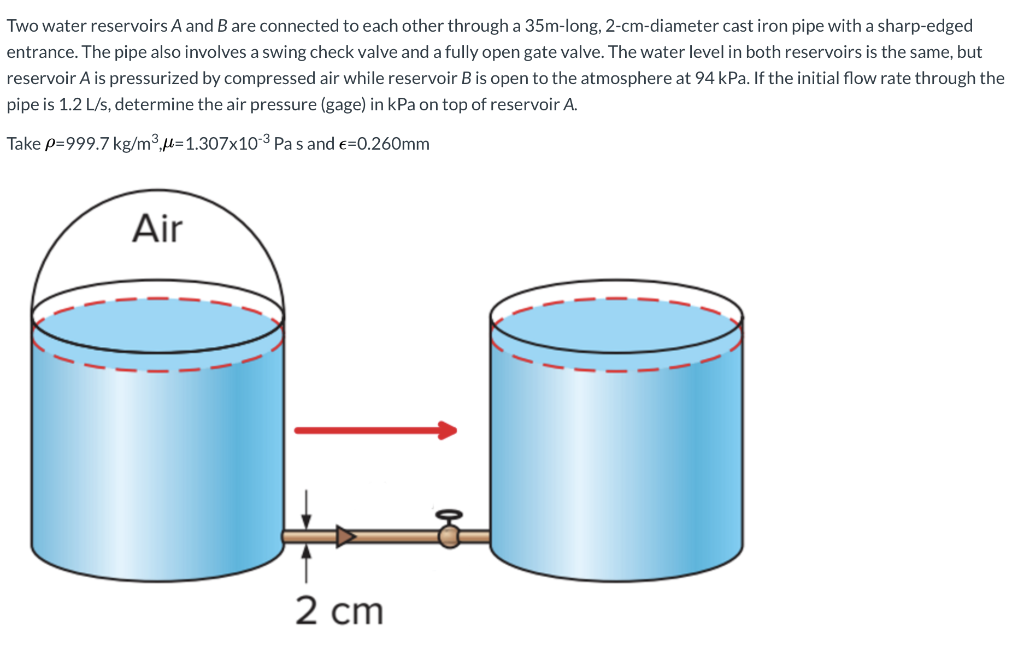Two water reservoirs A and B are connected to each other through a 35m-long, 2-cm-diameter cast iron pipe with a sharp-edged entrance. The pipe also involves a swing check valve and a fully open gate valve. The water level in both reservoirs is the same, but reservoir A is pressurized by compressed air while reservoir B is open to the atmosphere at 94 kPa. If the initial flow rate through the pipe is 1.2 L/s, determine the air pressure (gage)...

• Water flows from one large reservoir to another via a pipeline which is 0.9 m in...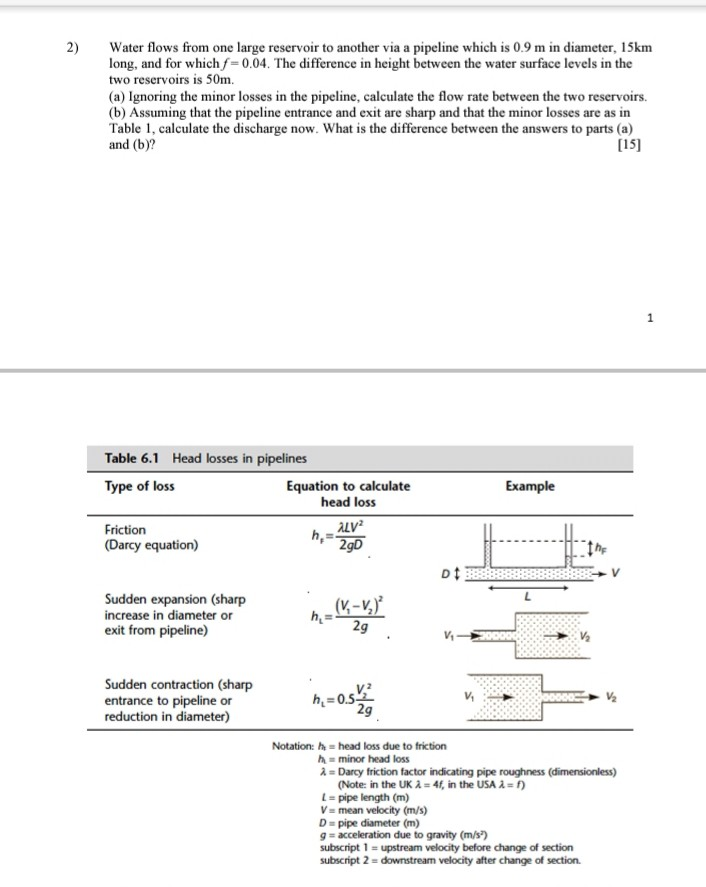Water flows from one large reservoir to another via a pipeline which is 0.9 m in diameter, 15km long, and for which f-0.04. The difference in height between the water surface levels in the two reservoirs is 50m. (a) Ignoring the minor losses in the pipeline, calculate the flow rate between the two reservoirs. (b) Assuming that the pipeline entrance and exit are sharp and that the minor losses are as in Table 1, calculate the discharge now. What is...

• 1. Water is pumped from a low reservoir to a high reservoir that is 20 m...

1. Water is pumped from a low reservoir to a high reservoir that is 20 m higher in elevation. The water is flowing at 1.5 m/s through a 305 mm internal diameter piping system. The water is at 18 degrees C. There are 1,000 m of piping, six standard 45 degree bends, two standard 90 degree bends, a branch flow tee, three gate valves, a swing check valve, a square inlet and a square outlet. The piping and valves are...